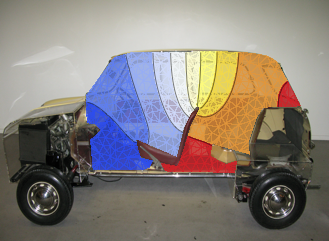# Differential EigensystemsMathematica Version 11 extends its symbolic and numerical differential equation-solving capabilities to include finding eigenvalues and eigenfunctions over regions. Given a possibly coupled partial differential equation (PDE), a region specification, and, optionally, boundary conditions, the eigensolvers find corresponding eigenvalues and eigenfunctions of the PDE operator over the given domain. This functionality will enable you to gain a deeper understanding of your physics and engineering designs.

## Key Features

 Find symbolic eigenvalues and eigenfunctions over regions. » Find numeric eigenvalues and eigenfunctions over regions. » Find eigenvalues and eigenfunctions of PDEs with arbitrary-order time derivative. Specify and solve eigenvalue problems in one, two, and three dimensions. Specify and solve constrained or unconstrained eigenvalue problems. » Freely formulate coupled PDEs for multi-physics eigenvalue analysis. » Specify domains using the full geometric region framework. » Use automatically generated meshes with curved boundaries for numeric eigenvalue computations. »